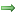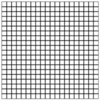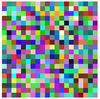# Lab Exercise for Assignment 5

## COMP112 2020 Tri 1: Lab Exercise 5

#### Goals

This assignment will give you more experience in writing programs that read some data from a file and do something with the data. It will also give you more practice using loops, especially nested loops for drawing grids.

#### Preparation

Download the zip file for lab exercise 5 and extract it to the `COMP112-2020T1-Lab5` folder in your home folder. It should contain templates for the exercises program. Read through the exercises and run the demo so that you know what you need to do.

Look at the model answers to assignment 4, and make sure you understand all the components of the programs. Also, go over the code examples from the lectures that used files.

#### Summary

Programs with files, loops, and conditions
• Image exercise:Two methods to draw grids of squares and random color squares.
• Files draw exercise:Series of programs to read data from files and draw the results.

To make a new color, you can use `new Color(r, g, b))` if `r`, `g`, and `b` are integers containing the red, green, and blue component values. `r`, `g`, and `b` must be between 0 and 255 (inclusive). If you put the new color into a variable (say, `colr`), you can set the UI to use that color with `UI.setColor(colr)`.

One way to obtain a random colour is to use the `Math.random` method:
```int r = (int)(Math.random()*256);   // random int between 0 and 255
int g = (int)(Math.random()*256);
int b = (int)(Math.random()*256);
Color color = new Color(r, g, b);
```

### Exercise Series 1: Drawing images

The first series of exercises is in the `ImageExercise` class. You are to complete three methods for drawing images. Each method involves a nested loop.

• `drawGridBoard` draws a grid of squares, the basic pattern for many board games.• `drawRandomImage` draws an image consisting of squares of random colours.### Exercise Series 2: Reading files for Drawing

The second series of exercises is in the `FilesDrawExercise` class. It contain two methods that each involve reading some data from a file and doing something with the data. Because each exercise addresses different kinds of file structure, you may find it helpful to do all the methods. Both methods are related to the `ImageDisplayer` assignment!

• `drawCircles` should read a file called circle-positions.txt containing pairs of numbers. It should draw a circle of diameter 30 at each position that it reads from the file. For example, if a line of the file has 120 350 on it, then it should draw a circle centered at (120, 350). You can make them all the same colour or random colours.

• `drawNames` should read a file of names and their positions called names.txt and draw them on the graphics pane. The first lot of names should be drawn in red, and the rest of the names should be drawn in green. The file has a header: the first token in the file is the number of names that should be displayed in red, the remaining names should be displayed in green. The rest of the file has triples of tokens: each triple has a name and two numbers.